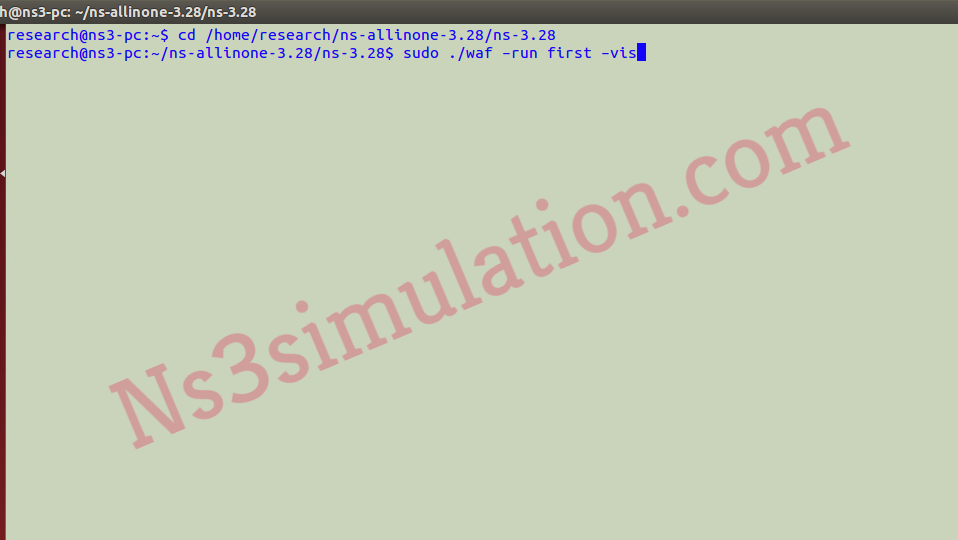Ns3 Projects for B.E/B.Tech M.E/M.Tech PhD Scholars.  Phone-Number:9790238391   E-mail: ns3simulation@gmail.com

# First.CC Ns3 Explanation

Do you guys need the best explanation about first.cc ns3 and research guidance based on ns3? Then, this article will guide you because we have enlisted the step by step processes that are required.

Phase: I

Initially, we have to install the ns-3.26 packages through the utilization of the below mentioned commands.

sudo ./waf build

Phase: II

Consequently, the performance based on simulation is file creation and that is stored in scratch folder in the name of first.cc. In addition, we have highlighted some sample code in the following.

• UDP packet transmission in first.cc
``` OnOffHelper myClientA ("ns3::UdpSocketFactory", destA); myClientA.SetAttribute ("OnTime", StringValue ("ns3::ConstantRandomVariable[Constant=1]")); myClientA.SetAttribute ("OffTime", StringValue ("ns3::ConstantRandomVariable[Constant=0]")); myClientA.SetAttribute ("DataRate", StringValue("100000kb/s")); myClientA.SetAttribute ("PacketSize", UintegerValue(payloadSize)); ApplicationContainer clientAppA = myClientA.Install (wifiStaNode.Get (0)); clientAppA.Start (Seconds (1.0)); clientAppA.Stop (Seconds (simulationTime + 1)); ```
• Position allocation in first.cc
``` //Set position for APs positionAlloc->Add (Vector (0.0, 0.0, 0.0)); positionAlloc->Add (Vector (30.0, 0.0, 0.0)); positionAlloc->Add (Vector (60.0, 0.0, 0.0)); positionAlloc->Add (Vector (90.0, 0.0, 0.0)); //Set position for STAs positionAlloc->Add (Vector (distance, 30.0, 0.0)); positionAlloc->Add (Vector (30 + distance, 30.0, 0.0)); positionAlloc->Add (Vector (60 + distance, 30.0, 0.0)); positionAlloc->Add (Vector (90 + distance, 30.0, 0.0)); ```
• MAC configuration in first.cc
``` ssid = Ssid ("network-A"); phy.Set ("ChannelNumber", UintegerValue(36)); mac.SetType ("ns3::StaWifiMac", "QosSupported", BooleanValue (true), "Ssid", SsidValue (ssid)); staDeviceA = wifi.Install (phy, mac, wifiStaNode.Get(0)); mac.SetType ("ns3::ApWifiMac", "QosSupported", BooleanValue (true), "Ssid", SsidValue (ssid), "BeaconGeneration", BooleanValue (true)); apDeviceA = wifi.Install (phy, mac, wifiApNode.Get(0)); ```

Phase: III

Then, we have to implement the main file through the execution of the following commands.

``` cd /home/research/ns-allinone-3.28/ns-3.28 sudo ./waf –run first –vis ```In addition, we have highlighted the results based on this command execution.